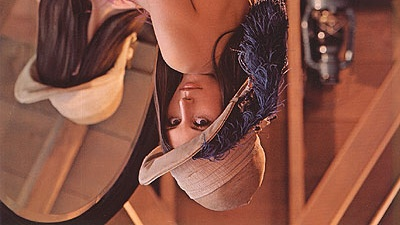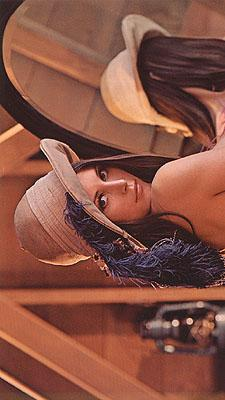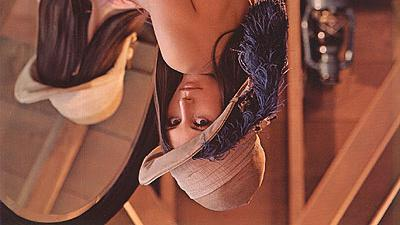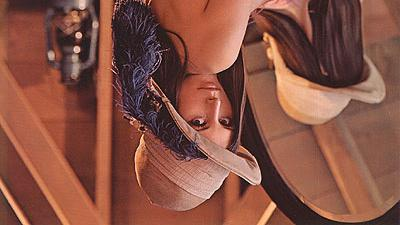# OpenCV, NumPy: Rotate and flip image

Posted: 2019-06-25 / Tags: Python, OpenCV, NumPy, Image Processing

Python's OpenCV handles images as NumPy array `ndarray`. There are functions for rotating or flipping images (= `ndarray`) in OpenCV and NumPy, either of which can be used.

Here, the following contents will be described.

• Rotate image with OpenCV: `cv2.rotate()`
• Flip image with OpenCV: `cv2.flip()`
• Rotate image with NumPy: `np.rot90()`
• Flip image with NumPy: `np.flip()`

For the basics of image processing with NumPy, see the following article that describes how to read an image file with Pillow(PIL) and convert it to `ndarray` without using OpenCV, and how to save `ndarray` as an image file.

For reading and writing images with OpenCV, see the following article:

You can also use Pillow (PIL) to rotate and flip an image.

Using Pillow is the simplest and easiest way if you just load an existing image file, rotate, flip and save it. It can rotate at any angle, not in 90 degree increments.

The sample code uses the following image as an example.## Rotate image with OpenCV: cv2.rotate()

The OpenCV function that rotates the image (= `ndarray`) is `cv2.rotate()`.

Specify the original `ndarray` as the first argument and the constant indicating the rotation angle and direction as the second argument `rotateCode`.

The following three constants can be specified in `rotateCode`.

• `cv2.ROTATE_90_CLOCKWISE`
• `cv2.ROTATE_90_COUNTERCLOCKWISE`
• `cv2.ROTATE_180`

Sample code and results are below.

``````import cv2

print(type(img))
# <class 'numpy.ndarray'>

print(img.shape)
# (225, 400, 3)

img_rotate_90_clockwise = cv2.rotate(img, cv2.ROTATE_90_CLOCKWISE)
cv2.imwrite('data/dst/lena_cv_rotate_90_clockwise.jpg', img_rotate_90_clockwise)
# True

img_rotate_90_counterclockwise = cv2.rotate(img, cv2.ROTATE_90_COUNTERCLOCKWISE)
cv2.imwrite('data/dst/lena_cv_rotate_90_counterclockwise.jpg', img_rotate_90_counterclockwise)
# True

img_rotate_180 = cv2.rotate(img, cv2.ROTATE_180)
cv2.imwrite('data/dst/lena_cv_rotate_180.jpg', img_rotate_180)
# True
``````## Flip image with OpenCV: cv2.flip()

The OpenCV function that flips the image (= `ndarray`) vertically and horizontally is `cv2.flip()`.

Specify the original `ndarray` as the first argument and a value indicating the directions the second argument `flipCode`.

The image is flipped according to the value of `flipCode` as follows:

• `flipcode = 0`: flip vertically
• `flipcode > 0`: flip horizontally
• `flipcode < 0`: flip vertically and horizontally

Sample code and results are below.

``````import cv2

print(type(img))
# <class 'numpy.ndarray'>

print(img.shape)
# (225, 400, 3)

img_flip_ud = cv2.flip(img, 0)
cv2.imwrite('data/dst/lena_cv_flip_ud.jpg', img_flip_ud)
# True

img_flip_lr = cv2.flip(img, 1)
cv2.imwrite('data/dst/lena_cv_flip_lr.jpg', img_flip_lr)
# True

img_flip_ud_lr = cv2.flip(img, -1)
cv2.imwrite('data/dst/lena_cv_flip_ud_lr.jpg', img_flip_ud_lr)
# True
``````## Rotate image with NumPy: np.rot90()

The NumPy function that rotates `ndarray` is `np.rot90()`.

Specify the original `ndarray` as the first argument, and the number of times to rotate 90 degrees as the second argument.

When the second argument is omitted, the default rotation is 90 degrees counterclockwise, and when the second argument is `2` and `3`, the rotation is 180 degrees and 270 degrees counterclockwise. See the following articles for details.

Sample code and results are below.

``````import numpy as np
from PIL import Image

img = np.array(Image.open('data/src/lena.jpg'))
print(type(img))
# <class 'numpy.ndarray'>

print(img.shape)
# (225, 400, 3)

Image.fromarray(np.rot90(img)).save('data/dst/lena_np_rot90.jpg')

Image.fromarray(np.rot90(img, 2)).save('data/dst/lena_np_rot90_180.jpg')

Image.fromarray(np.rot90(img, 3)).save('data/dst/lena_np_rot90_270.jpg')
``````Here, the image file is read and written by using Pillow(PIL), but when reading and writing with OpenCV as in the example above, you can also use the NumPy function instead of the OpenCV function to process the rotation. Both are just rotating `ndarray`.

The same is true for the following example for flipping.

## Flip image with NumPy: np.flip()

The NumPy function that flips `ndarray` vertically and horizontally is `np.flip()`. There are also `np.flipud()` which flips vertically (up and down) and `np.fliplr()` which flips horizontally (left and right).

You can flip `ndarray` only vertically or horizontally by specifying an argument of `np.flip()`, but it is easier to use `np.flipud()` and `np.fliplr()`.

If you want to flip `ndarray` both vertically and horizontally, you need to use `np.flip ()`. See the following article for details.

Sample code and results are below.

``````import numpy as np
from PIL import Image

img = np.array(Image.open('data/src/lena.jpg'))
print(type(img))
# <class 'numpy.ndarray'>

print(img.shape)
# (225, 400, 3)

Image.fromarray(np.flipud(img)).save('data/dst/lena_np_flipud.jpg')

Image.fromarray(np.fliplr(img)).save('data/dst/lena_np_fliplr.jpg')

Image.fromarray(np.flip(img, (0, 1))).save('data/dst/lena_np_flip_ud_lr.jpg')
``````